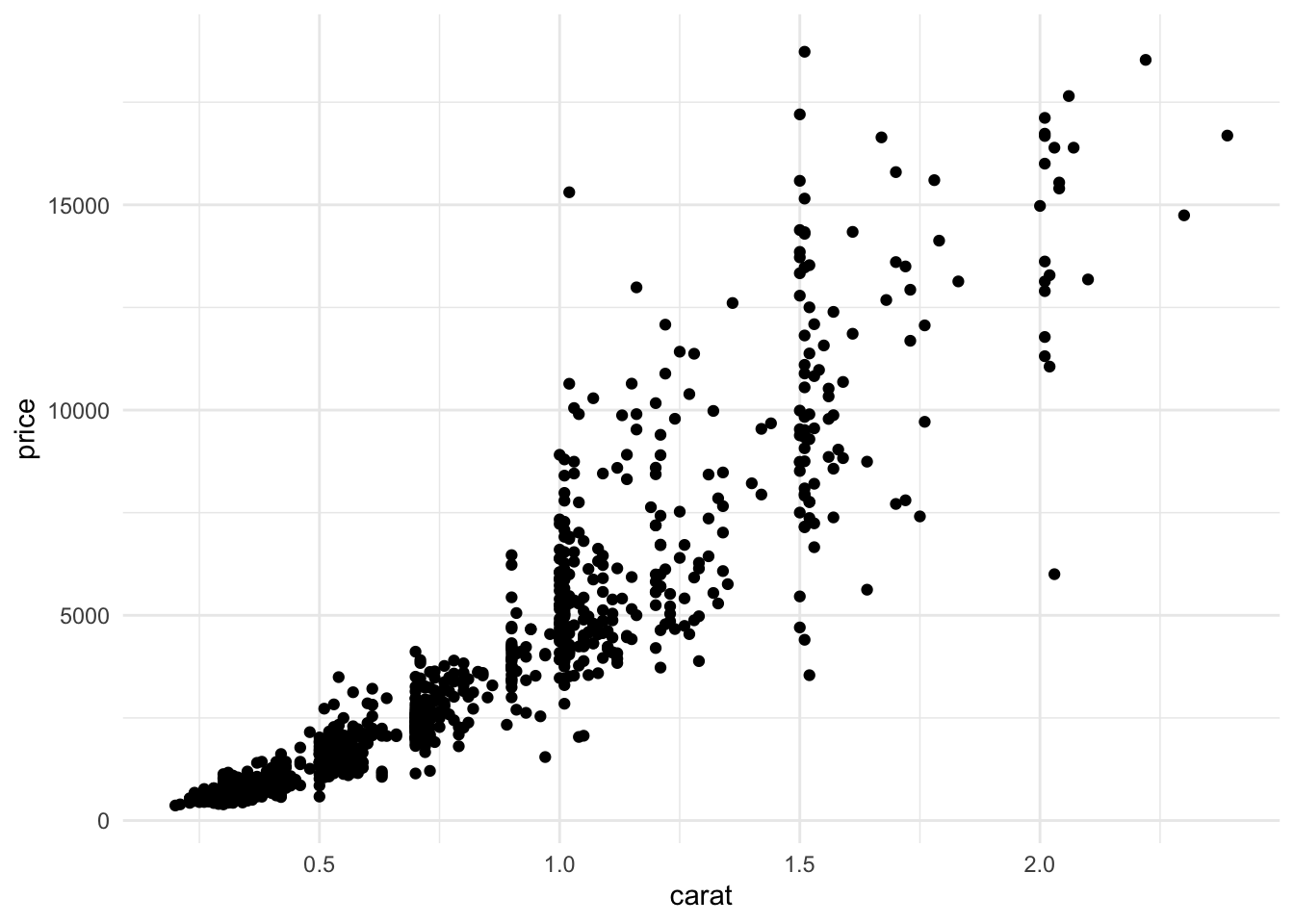## Diamonds

Here, we will apply the methods shown in the notes to a data set of diamonds. This is a classic statistics data set used in a lot of notes that you may find online. Our variable of interest is the price of each diamond.

``````set.seed(1)

diamond <- read_csv(file.path("data", "diamonds.csv")) %>%
mutate(train_id = if_else(runif(n()) < 0.6, "train", "valid"))
diamond``````
``````## # A tibble: 1,000 x 11
##    price carat cut       color clarity depth table     x     y     z train_id
##    <dbl> <dbl> <chr>     <chr> <chr>   <dbl> <dbl> <dbl> <dbl> <dbl> <chr>
##  1   787 0.28  Ideal     F     VVS2     62.6    55  4.2   4.23  2.64 valid
##  2  2346 0.71  Very Good F     SI1      62.1    59  5.64  5.73  3.53 valid
##  3   478 0.26  Ideal     G     VS1      62.1    55  4.09  4.12  2.55 train
##  4  6389 1     Good      D     VS2      64.1    58  6.24  6.33  4.03 train
##  5  5818 1.2   Good      I     VS1      59.6    61  6.84  6.89  4.09 train
##  6  1369 0.570 Very Good G     SI1      63.4    57  5.26  5.21  3.32 valid
##  7  2278 0.75  Ideal     E     SI2      62.1    57  5.81  5.85  3.62 train
##  8   570 0.3   Ideal     F     VS1      62.6    57  4.29  4.34  2.7  valid
##  9  2980 0.64  Good      F     IF       58.6    61  5.69  5.71  3.34 train
## 10  2854 0.73  Premium   E     VS2      62      57  5.86  5.76  3.6  valid
## # … with 990 more rows``````

Start by creating a scatter plot with carat on the x-axis and price on the y-axis.

``````diamond %>%
ggplot(aes(carat, price)) +
geom_point()``````Before we start building predictive models, it is good to have a baseline for a good RMSE. Compute the RMSE on the train and valid sets using a model that just takes the mean value of `price`.

``````diamond %>%
mutate(pred_const = mean(price)) %>%
group_by(train_id) %>%
summarize(rmse = sqrt(mean((price - pred_const)^2)))``````
``````## # A tibble: 2 x 2
##   train_id  rmse
##   <chr>    <dbl>
## 1 train    3678.
## 2 valid    3565.``````

Now, buimd a model that predicts price as a function of a diamond’s carat on the training set. Calculate the RMSE for the training and validation sets.

``````model <- diamond %>%
filter(train_id == "train") %>%
lm(price ~ carat, data = .)

diamond %>%
mutate(pred_lm = predict(model, newdata = .)) %>%
group_by(train_id) %>%
summarize(rmse = sqrt(mean((price - pred_lm)^2)))``````
``````## # A tibble: 2 x 2
##   train_id  rmse
##   <chr>    <dbl>
## 1 train    1433.
## 2 valid    1545.``````

These should be almost half the size of the RMSE you had for the constant model.

Now, add color (a categorical variable) into the model and compute the RMSE.

``````model <- diamond %>%
filter(train_id == "train") %>%
lm(price ~ carat + color, data = .)

diamond %>%
mutate(pred_lm = predict(model, newdata = .)) %>%
group_by(train_id) %>%
summarize(rmse = sqrt(mean((price - pred_lm)^2)))``````
``````## # A tibble: 2 x 2
##   train_id  rmse
##   <chr>    <dbl>
## 1 train    1365.
## 2 valid    1481.``````

Does the RMSE improve? Answer: Yes, by a little bit.

Now, print a summary of the model you just created.

``summary(model)``
``````##
## Call:
## lm(formula = price ~ carat + color, data = .)
##
## Residuals:
##     Min      1Q  Median      3Q     Max
## -5311.0  -771.6   -33.7   605.9  7403.1
##
## Coefficients:
##             Estimate Std. Error t value Pr(>|t|)
## (Intercept) -2322.78     177.62 -13.077  < 2e-16 ***
## carat        8004.62     133.34  60.032  < 2e-16 ***
## colorE        -72.20     198.54  -0.364   0.7162
## colorF        115.70     202.27   0.572   0.5675
## colorG         89.04     197.55   0.451   0.6524
## colorH       -399.59     215.18  -1.857   0.0638 .
## colorI       -992.23     241.20  -4.114 4.44e-05 ***
## colorJ      -1878.43     346.56  -5.420 8.64e-08 ***
## ---
## Signif. codes:  0 '***' 0.001 '**' 0.01 '*' 0.05 '.' 0.1 ' ' 1
##
## Residual standard error: 1374 on 599 degrees of freedom
## Multiple R-squared:  0.8622, Adjusted R-squared:  0.8606
## F-statistic: 535.4 on 7 and 599 DF,  p-value: < 2.2e-16``````

For a given size, what color seems to be the most expensive? Answer F.

Now, fit a model that uses carat and clarity to predict the price of a diamond. Compute the RMSE of this model and compare to the previous two models.

``````model <- diamond %>%
filter(train_id == "train") %>%
lm(price ~ carat + clarity, data = .)

diamond %>%
mutate(pred_lm = predict(model, newdata = .)) %>%
group_by(train_id) %>%
summarize(rmse = sqrt(mean((price - pred_lm)^2)))``````
``````## # A tibble: 2 x 2
##   train_id  rmse
##   <chr>    <dbl>
## 1 train    1187.
## 2 valid    1268.``````

Modify the previous model to include a different slope and interecept for each clarity. Again, compute the RMSE.

``````model <- diamond %>%
filter(train_id == "train") %>%
lm(price ~ carat * clarity, data = .)

diamond %>%
mutate(pred_lm = predict(model, newdata = .)) %>%
group_by(train_id) %>%
summarize(rmse = sqrt(mean((price - pred_lm)^2)))``````
``````## # A tibble: 2 x 2
##   train_id  rmse
##   <chr>    <dbl>
## 1 train    1125.
## 2 valid    1183.``````

Takign the model from the previous question, predict prices for all of the diamands and plot the fit on a plot with carat on the x-axis, price on the y-axis, and lines colored by clarity. Verify that the lines have different slopes and intercepts.

``````diamond %>%
mutate(pred_lm = predict(model, newdata = .)) %>%
ggplot(aes(carat, price)) +
geom_point(aes(color = clarity), alpha = 0.2) +
geom_line(aes(y = pred_lm, color = clarity))``````